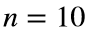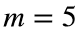# Problem 51950. Find the smallest integer m such that n divides m!

Write a function that takes an integer n and finds the smallest integer m whose factorial is divisible by n. For example, if, then the smallest factorial that is a multiple of n is 5! = 120; therefore,.

### Solution Stats

42.11% Correct | 57.89% Incorrect
Last Solution submitted on Jun 01, 2023

### Community Treasure Hunt

Find the treasures in MATLAB Central and discover how the community can help you!

Start Hunting!